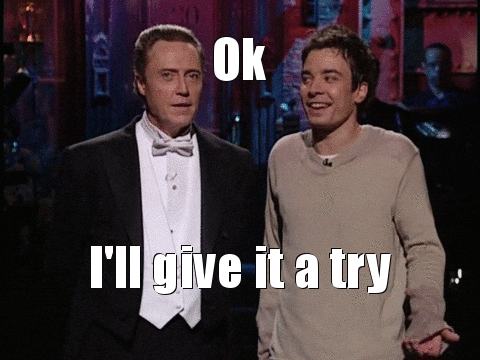## DEV CommunityLiu Yongliang

Posted on • Updated on

# Compile-Time Type Vs Run-Time Type - [OOP & Java #3]

I have a Paper object that contains 2 `equals` method.

``````class Paper{
private int height;
private int width;

Paper(int height, int width){
this.height= height;
this.width = width;
}
@Override
public boolean equals(Object obj){
System.out.println("A");
return this == obj;
}
public boolean equals(Paper p){
System.out.println("B");
return this == p;
}
}
``````

What will be printed out for the following 6 `equals` method calls?

``````//set up
Paper paper = new Paper(297, 140);
Object object = paper;
// 1 to 3
object.equals( object );
object.equals( (Paper) object );
object.equals( paper );
// 4 to 6
paper.equals( object );
paper.equals( (Paper) object );
paper.equals( paper );
````````````// 1 to 3
object.equals( object );         // A
object.equals( (Paper) object ); // A
object.equals( paper );          // A
// 4 to 6
paper.equals( object );           // A
paper.equals( (Paper) object );   // B
paper.equals( paper );            // B
``````

Let's get to the analysis. Feel free to correct me if I am wrong...

### Basic knowledge

Compile-time Run-time
Type Left hand side of the assignment,
fixed upon declaration
Right hand side of the assignment,
vary as program runs
Binding Static Dynamic
Control Restricts methods that can be called based on compile-time type Methods to invoke are determined during run-time
more specific method will be invoked
Method overriding,
overridden method will never be invoked

There are a total of 3 different possible outputs :

• None (Java Object's default equals method is invoked)
• A (Paper's `equals` overriding method is invoked)
• B (Paper's `equals` overloading method is invoked)

Explanation for the set-up

``````//set up
Paper paper = new Paper(297, 140);
Object object = paper;
``````
• A variable named `paper` is created and declared to be of compile-time type `Paper`. The variable is also assigned to a new `Paper` object created using the `Paper` class constructor. The run-time type of the variable is also `Paper`.
• A variable named `object` is created and declared to be of compile-time type `Object`. The variable is also assigned to the created `Paper` object. The run-time type of the variable `object` is `Paper`.

Explanation for the 1st to 3rd method calls

``````// 1 to 3
object.equals( object );         // A
object.equals( (Paper) object ); // A
object.equals( paper );          // A
``````

During compile-time, we look at the compile-time type. So in the above three cases, we have a variable named `object` that has compile-time type of `Object`. This fact tells us that the variable can execute any method as long as the method is included in the `Object` class.

At the same time, we check if there is any method overloading, where two or more methods have the same name but different method signatures. If there is method overloading, we will look for the most specific method within that class to call upon. This is static binding.

During run-time, we know that certain objects might have different type as compared to compile-time, hence we check if any method has been overridden and change our decision made during compile-time, and run the overriding method instead. This is also known as dynamic binding.

From our mental model above, we can see that the `Object` class has only one `equals` method. So,

• Method call 1 is safe. During compile-time, we loosely understand method call 1 as `(Object) object.equals( (Object) object )`. Since we do have the `equals` method within `Object` class, we know at the very least, `equals` method can be called from within `Object` class. Come run-time, we realize that object has a run-time type of `Paper`. We now check for any override (not overload!), and turns out that there is an overriding `equals` method within the child `Paper` class and hence that method is invoked and printed "A".
• Method call 2 is safe. We can see that due to typecasting, a `Paper` object is being passed into the `equals` method. Although we do not have a particular `equals` method in Object class that takes in `Paper`, we can treat the parameter as `Object`. This is because `Paper` is a subclass of `Object` (by default, all objects in Java inherit from `Object`). Hence, following the same logical reasoning of method call 1, the `equals` method within `Paper` class is invoked and printed "A".
• Method call 3 is safe. Reasoning follows method call 2.

• The ability to invoke the overriding method in the subclass instead of the overridden method in the parent class supports polymorphism. This means given objects of the same parent, we can call a particular method and invoke different implementations of that method within each child class.

Explanation for the 4th to 6th method calls

``````// 4 to 6
paper.equals( object );           // A
paper.equals( (Paper) object );   // B
paper.equals( paper );            // B
``````

With the knowledge from the first three method calls, it is much easier to understand the rest.

• Method call 4 is safe. We do have an `equals` method within the `Paper` class and looking at the method signature, we know that we will call the one that specifically takes in `Object`, which outputs "A".
• Method call 5 is safe. `(Paper)object` signals that we want the object to be used as `Paper` when this method is run. During compile-time, We see that the parameter is typecast into `Paper`, so we look for a specific method call that match this. We have the second `equals` method within `Paper` that specify that it takes in `Paper` and outputs "B".
• Method call 6 is safe. Reasoning is similar to method call 5.

• The only time that the overloaded method `equals(Paper)` can be invoked is when the compile-time type of the variable is `Paper`.